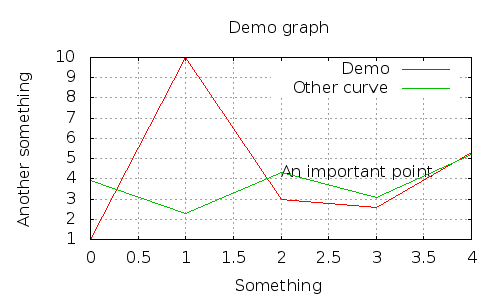GnuPlot library - forked from gregwar/gnuplot

##### Details

Homepage

Source

35

0

0

0

11

0.1.5 2015-03-12 09:29 UTC

This package is auto-updated.

Last update: 2023-01-10 03:30:32 UTC

A PHP Library for using GnuPlot

This is the output of the `demo/write.php`:# Requirements

You need to have a server with `gnuplot` installed and the safe mode disabled (to be able to run `proc_open()`)

# Usage

There is examples in the `demo/` directory.

You can create a graph and populate it like this:

```<?php

use Gregwar\GnuPlot\GnuPlot;

\$plot = new GnuPlot;

// Setting the main graph title
\$plot->setGraphTitle('Demo graph');

// Adding three points to the first curve
\$plot
->setTitle(0, 'The first curve')
->push(0, 4)
->push(1, 5)
->push(2, 6)
;

// Adding three points on the other curve and drawing it as a line of connected points, colored in red and smoothed
// (with index 1)
\$plot
->setTitle(1, 'The first curve')
->setLineType(1, 'rgb #ff0000')
->setLineMode(1, 'lp')
->setLineSmooth(1, GnuPlot::SMOOTH_CSPLINE)
->push(0, 8, 1)
->push(1, 9, 1)
->push(2, 10, 1)
;

// Drawing the area between the two curves in blue
\$plot
->setLineMode(2, GnuPlot::LINEMODE_FILLEDCURVES)
->setLineType(2, 'rgb #0000ff')
->setTitle(2, 'Area')
->push(0, [4, 8], 2)
->push(1, [5, 9], 2)
->push(2, [6,10], 2)
;```

You can then save it to a file, have a look to `write.php` for example:

```<?php

// Write the graph to out.png
\$plot->writePng('out.png');```

Or render it directly into a browser, you can try `out.php` for example:

```<?php

echo \$plot->get();```

Or display it on the screen (useful with CLI scripts), run the `demo.php` script for example:

```<?php

\$plot->display();```

Or display it, and re-feed it in real time (with CLI scripts), you can run `realTime.php` for example:

```<?php

\$plot->refresh();```

# API

• `push(\$x, \$y, \$index=0)`, add a point to the \$index-nth curve (\$y can be an array if the linemode is `GnuPlot::LINEMODE_FILLEDCURVES`)
• `display()`, renders the graph on the screen (asuming you are using it as a CLI with an X Server
• `refresh()`, same as `display()`, but will replot the graph after the first call
• `get()`, gets the PNG data for your image
• `writePng(\$filename)`, writes the data to the output PNG file
• `writePDF(\$filename)`, writes the data to the output PDF file
• `writeEPS(\$filename)`, writes the data to the output EPS file
• `setTitle(\$index, \$title)`, sets the title of the \$index-nt curve
• `setLineWidth(\$index, \$width)`, sets the width of the \$index-nt curve
• `setLineMode(\$index, \$mode)`, sets the line mode of the \$index-nt curve (set to `GnuPlot::LINEMODE_FILLEDCURVES` to fill an area between two lines)
• `setLinePoint(\$index, \$point)`, sets the line point of the \$index-nt curve
• `setLineType(\$index, \$type)`, sets the line type of the \$index-nt curve
• `setLineColor(\$index, \$color)`, sets the line color of the \$index-nt curve
• `setLineSmooth(\$index, \$smooth)`, sets the smooth type of the \$index-nt curve. Available smooths are `SMOOTH_NONE`, `SMOOTH_BEZIER`, `SMOOTH_CSPLINE`, defined as constants on the `GnuPlot` class.
• `setGraphTitle(\$title)`, sets the main title for the graph
• `setXTimeFormat(\$format)`, sets the X axis as a time axis and specify data format
• `setTimeFormatString(\$format)`, specify the X axis time presentation format
• `setXLabel(\$text)`, sets the label for the X axis
• `setYLabel(\$text)`, sets the label for the Y axis
• `setYFormat(\$format)`, sets Y axis formatting
• `setXRange(\$min, \$max)`, set the X min & max
• `setYRange(\$min, \$max)`, set the Y min & max
• `setXTics(\$tics)`, set the X tics
• `setYTics(\$tics)`, set the Y tics
• `setMXTics(\$tics)`, set the micro X tics
• `setMYTics(\$tics)`, set the micro Y tics
• `setMinorGrid(\$status)`, enabled/disables the grid for microtics
• `setGridPlacement(\$layer)`, sets the placement of the grid, can be `GnuPlot::GRID_DEFAULT`, `GnuPlot::GRID_FRONT` or `GnuPlot::GRID_BACK`
• `setWidth(\$width)`, sets the width of the graph
• `setHeight(\$height)`, sets the height of the graph
• `setCanvasWidth(\$width)`, sets the width of the canvas (if not set, the width value is used)
• `setCanvasHeight(\$height)`, sets the height of the canvas (if not set, the height value is used)
• `setOrigin(\$x, \$y)`, sets the origin of the graph
• `setSleepTime(\$sleepTime)`, sets the sleep time after saving a file
• `addLabel(\$x, \$y, \$text)`, add some label at a point
• `flush()`, completely flushes the internal state and resets the object to its initial state

`Gregwar\GnuPlot` is under MIT license